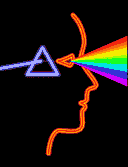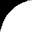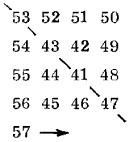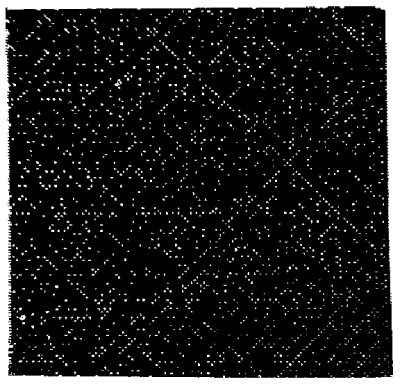Science Frontiers ONLINE No. 42: Nov-Dec 1985Issue Contents

### Other pages### The Fabric Of Prime Number Distribution

Mathematicians and many non-mathematicians have soft spots in their hearts for numbers that cannot be subdivided; that is, the prime numbers. No one has ever been able to figure out any foolproof system to their occurrence or how to generate them all by formula. Some primes, such as 11 and 13, 17 and 19, etc., come in pairs; but most don't. And some formulas are particularly good at generating primes, but they all fail somewhere. One such formula was discovered by Leonhard Euler, the great mathematician: n2 + n + 41 This formula works for n = 0, 1, 2,...39; but fails at n = 40.

An interesting consequence of Euler's formula can be made apparent when all numbers from 41 to 440 are written in a square spiral, like so:All of the numbers on the diagonal indicated are Euler formula primes, even when the spiral is expanded to 20 x 20. However, when the 20 x 20 spiral is examined closely, many of the other primes -- those not generated by Euler's formula -- also tend to line up on diagonals. This is a most intriguing characteristic, one which goes far beyond the 20 x 20 array mentioned above. The computer-generated display shown below lays out a huge square spiral, with each prime a bright dot. The picture has a pronounced diagonal texture. Why this is so is a mathematical mystery. (Crypton, Dr.; "Prime Numbers and National Security, " Science Digest, 93: 86, October 1985. ) (Does the diagonal fabric of primes have any practical significance ? At the moment no one knows. Again and again, abstruse mathematical structures have turned out to mirror phenomena in the world we call "real". WRC)From Science Frontiers #42, NOV-DEC 1985. © 1985-2000 William R. Corliss